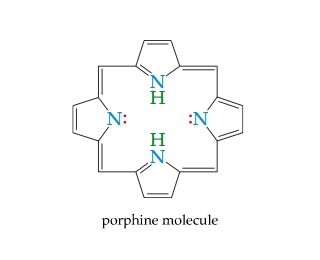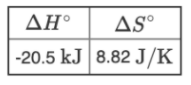# Problem: The porphine molecule(Figure 2) is a tetradentate ligand and is the basis for many porphyrins found in biological systems. Porphyrins are complexes with metal ions and have different substituents attached to the carbons of the porphine ligand. Heme (which contains Fe2+) and chlorophyll (which contains Mg2+) are two common examples.M(H2O)mn+(aq) + por(aq) ⇌ M(por)n+(aq) + mH2O(l)Based on the values of standard enthalpy, ΔH°, and entropy, ΔS°, shown here,what is the value of the formation constant, Kf, for the reaction at 25°C?

###### FREE Expert Solution

We’re being asked to determine the equilibrium constant (K) at 298 K for the given reaction:

M(H2O)mn+(aq) + por(aq) ⇌ M(por)n+(aq) + mH2O(l)

Recall that ΔG˚rxn and K are related to each other:

$\overline{){\mathbf{\Delta G}}{{\mathbf{°}}}_{{\mathbf{rxn}}}{\mathbf{=}}{\mathbf{-}}{\mathbf{RTlnK}}}$

We can use the following equation to solve for ΔG˚rxn:

$\overline{){\mathbf{\Delta G}}{{\mathbf{°}}}_{{\mathbf{rxn}}}{\mathbf{=}}{\mathbf{\Delta H}}{{\mathbf{°}}}_{{\mathbf{rxn}}}{\mathbf{-}}{\mathbf{T\Delta S}}{{\mathbf{°}}}_{{\mathbf{rxn}}}}$

For this problem, we need to do the following steps:

Step 1: Use ΔH˚rxn and ΔS˚rxn to calculate for ΔG˚rxn.

Step 2: Calculate for Kf

92% (82 ratings)###### Problem Details

The porphine molecule(Figure 2) is a tetradentate ligand and is the basis for many porphyrins found in biological systems. Porphyrins are complexes with metal ions and have different substituents attached to the carbons of the porphine ligand. Heme (which contains Fe2+) and chlorophyll (which contains Mg2+) are two common examples.M(H2O)mn+(aq) + por(aq) ⇌ M(por)n+(aq) + mH2O(l)

Based on the values of standard enthalpy, ΔH°, and entropy, ΔS°, shown here,what is the value of the formation constant, Kf, for the reaction at 25°C?